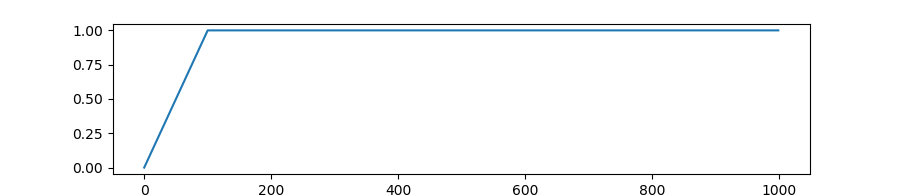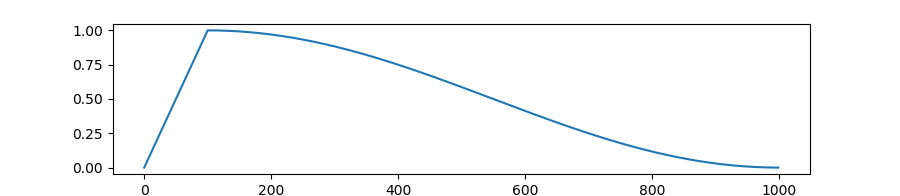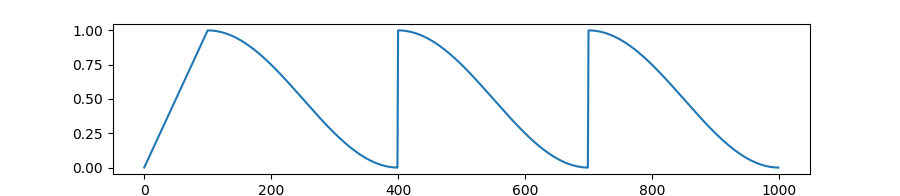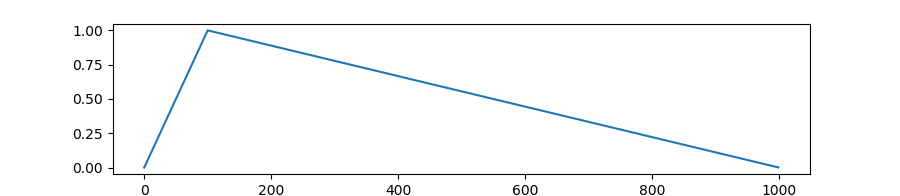# Optimization¶

The .optimization module provides:

• an optimizer with weight decay fixed that can be used to fine-tuned models, and

• several schedules in the form of schedule objects that inherit from _LRSchedule:

• a gradient accumulation class to accumulate the gradients of multiple batches

## AdamW (PyTorch)¶

class transformers.AdamW(params: Iterable[torch.nn.parameter.Parameter], lr: float = 0.001, betas: Tuple[float, float] = 0.9, 0.999, eps: float = 1e-06, weight_decay: float = 0.0, correct_bias: bool = True)[source]

Implements Adam algorithm with weight decay fix as introduced in Decoupled Weight Decay Regularization.

Parameters
• params (Iterable[torch.nn.parameter.Parameter]) – Iterable of parameters to optimize or dictionaries defining parameter groups.

• lr (float, optional, defaults to 1e-3) – The learning rate to use.

• betas (Tuple[float,float], optional, defaults to (0.9, 0.999)) – Adam’s betas parameters (b1, b2).

• eps (float, optional, defaults to 1e-6) – Adam’s epsilon for numerical stability.

• weight_decay (float, optional, defaults to 0) – Decoupled weight decay to apply.

• correct_bias (bool, optional, defaults to True) – Whether ot not to correct bias in Adam (for instance, in Bert TF repository they use False).

step(closure: Callable = None)[source]

Performs a single optimization step.

Parameters

closure (Callable, optional) – A closure that reevaluates the model and returns the loss.

## AdamWeightDecay (TensorFlow)¶

class transformers.AdamWeightDecay(learning_rate: Union[float, tensorflow.python.keras.optimizer_v2.learning_rate_schedule.LearningRateSchedule] = 0.001, beta_1: float = 0.9, beta_2: float = 0.999, epsilon: float = 1e-07, amsgrad: bool = False, weight_decay_rate: float = 0.0, include_in_weight_decay: Optional[List[str]] = None, exclude_from_weight_decay: Optional[List[str]] = None, name: str = 'AdamWeightDecay', **kwargs)[source]

Adam enables L2 weight decay and clip_by_global_norm on gradients. Just adding the square of the weights to the loss function is not the correct way of using L2 regularization/weight decay with Adam, since that will interact with the m and v parameters in strange ways as shown in Decoupled Weight Decay Regularization.

Instead we want ot decay the weights in a manner that doesn’t interact with the m/v parameters. This is equivalent to adding the square of the weights to the loss with plain (non-momentum) SGD.

Parameters
• learning_rate (Union[float, tf.keras.optimizers.schedules.LearningRateSchedule], optional, defaults to 1e-3) – The learning rate to use or a schedule.

• beta_1 (float, optional, defaults to 0.9) – The beta1 parameter in Adam, which is the exponential decay rate for the 1st momentum estimates.

• beta_2 (float, optional, defaults to 0.999) – The beta2 parameter in Adam, which is the exponential decay rate for the 2nd momentum estimates.

• epsilon (float, optional, defaults to 1e-7) – The epsilon paramenter in Adam, which is a small constant for numerical stability.

• amsgrad (bool, optional, default to False) – Wheter to apply AMSGrad varient of this algorithm or not, see On the Convergence of Adam and Beyond.

• weight_decay_rate (float, optional, defaults to 0) – The weight decay to apply.

• include_in_weight_decay (List[str], optional) – List of the parameter names (or re patterns) to apply weight decay to. If none is passed, weight decay is applied to all parameters by default (unless they are in exclude_from_weight_decay).

• exclude_from_weight_decay (List[str], optional) – List of the parameter names (or re patterns) to exclude from applying weight decay to. If a include_in_weight_decay is passed, the names in it will supersede this list.

• name (str, optional, defaults to ‘AdamWeightDecay’) – Optional name for the operations created when applying gradients.

• kwargs – Keyward arguments. Allowed to be {clipnorm, clipvalue, lr, decay}. clipnorm is clip gradients by norm; clipvalue is clip gradients by value, decay is included for backward compatibility to allow time inverse decay of learning rate. lr is included for backward compatibility, recommended to use learning_rate instead.

transformers.create_optimizer(init_lr: float, num_train_steps: int, num_warmup_steps: int, min_lr_ratio: float = 0.0, adam_epsilon: float = 1e-08, weight_decay_rate: float = 0.0, include_in_weight_decay: Optional[List[str]] = None)[source]

Creates an optimizer with a learning rate schedule using a warmup phase followed by a linear decay.

Parameters
• init_lr (float) – The desired learning rate at the end of the warmup phase.

• num_train_step (int) – The total number of training steps.

• num_warmup_steps (int) – The number of warmup steps.

• min_lr_ratio (float, optional, defaults to 0) – The final learning rate at the end of the linear decay will be init_lr * min_lr_ratio.

• adam_epsilon (float, optional, defaults to 1e-8) – The epsilon to use in Adam.

• weight_decay_rate (float, optional, defaults to 0) – The weight decay to use.

• include_in_weight_decay (List[str], optional) – List of the parameter names (or re patterns) to apply weight decay to. If none is passed, weight decay is applied to all parameters except bias and layer norm parameters.

## Schedules¶

### Learning Rate Schedules (Pytorch)¶

transformers.get_constant_schedule(optimizer: torch.optim.optimizer.Optimizer, last_epoch: int = - 1)[source]

Create a schedule with a constant learning rate, using the learning rate set in optimizer.

Parameters
• optimizer (Optimizer) – The optimizer for which to schedule the learning rate.

• last_epoch (int, optional, defaults to -1) – The index of the last epoch when resuming training.

Returns

torch.optim.lr_scheduler.LambdaLR with the appropriate schedule.

transformers.get_constant_schedule_with_warmup(optimizer: torch.optim.optimizer.Optimizer, num_warmup_steps: int, last_epoch: int = - 1)[source]

Create a schedule with a constant learning rate preceded by a warmup period during which the learning rate increases linearly between 0 and the initial lr set in the optimizer.

Parameters
• optimizer (Optimizer) – The optimizer for which to schedule the learning rate.

• num_warmup_steps (int) – The number of steps for the warmup phase.

• last_epoch (int, optional, defaults to -1) – The index of the last epoch when resuming training.

Returns

torch.optim.lr_scheduler.LambdaLR with the appropriate schedule.transformers.get_cosine_schedule_with_warmup(optimizer: torch.optim.optimizer.Optimizer, num_warmup_steps: int, num_training_steps: int, num_cycles: float = 0.5, last_epoch: int = - 1)[source]

Create a schedule with a learning rate that decreases following the values of the cosine function between the initial lr set in the optimizer to 0, after a warmup period during which it increases linearly between 0 and the initial lr set in the optimizer.

Parameters
• optimizer (Optimizer) – The optimizer for which to schedule the learning rate.

• num_warmup_steps (int) – The number of steps for the warmup phase.

• num_training_steps (int) – The total number of training steps.

• num_cycles (float, optional, defaults to 0.5) – The number of waves in the cosine schedule (the defaults is to just decrease from the max value to 0 following a half-cosine).

• last_epoch (int, optional, defaults to -1) – The index of the last epoch when resuming training.

Returns

torch.optim.lr_scheduler.LambdaLR with the appropriate schedule.transformers.get_cosine_with_hard_restarts_schedule_with_warmup(optimizer: torch.optim.optimizer.Optimizer, num_warmup_steps: int, num_training_steps: int, num_cycles: int = 1, last_epoch: int = - 1)[source]

Create a schedule with a learning rate that decreases following the values of the cosine function between the initial lr set in the optimizer to 0, with several hard restarts, after a warmup period during which it increases linearly between 0 and the initial lr set in the optimizer.

Parameters
• optimizer (Optimizer) – The optimizer for which to schedule the learning rate.

• num_warmup_steps (int) – The number of steps for the warmup phase.

• num_training_steps (int) – The total number of training steps.

• num_cycles (int, optional, defaults to 1) – The number of hard restarts to use.

• last_epoch (int, optional, defaults to -1) – The index of the last epoch when resuming training.

Returns

torch.optim.lr_scheduler.LambdaLR with the appropriate schedule.transformers.get_linear_schedule_with_warmup(optimizer, num_warmup_steps, num_training_steps, last_epoch=- 1)[source]

Create a schedule with a learning rate that decreases linearly from the initial lr set in the optimizer to 0, after a warmup period during which it increases linearly from 0 to the initial lr set in the optimizer.

Parameters
• optimizer (Optimizer) – The optimizer for which to schedule the learning rate.

• num_warmup_steps (int) – The number of steps for the warmup phase.

• num_training_steps (int) – The totale number of training steps.

• last_epoch (int, optional, defaults to -1) – The index of the last epoch when resuming training.

Returns

torch.optim.lr_scheduler.LambdaLR with the appropriate schedule.### Warmup (TensorFlow)¶

class transformers.WarmUp(initial_learning_rate: float, decay_schedule_fn: Callable, warmup_steps: int, power: float = 1.0, name: str = None)[source]

Applies a warmup schedule on a given learning rate decay schedule.

Parameters
• initial_learning_rate (float) – The initial learning rate for the schedule after the warmup (so this will be the learning rate at the end of the warmup).

• decay_schedule_fn (Callable) – The schedule function to apply after the warmup for the rest of training.

• warmup_steps (int) – The number of steps for the warmup part of training.

• power (float, optional, defaults to 1) – The power to use for the polynomial warmup (defaults is a linear warmup).

• name (str, optional) – Optional name prefix for the returned tensors during the schedule.

### GradientAccumulator (TensorFlow)¶
class transformers.GradientAccumulator[source]
Gradient accumulation utility. When used with a distribution strategy, the accumulator should be called in a replica context. Gradients will be accumulated locally on each replica and without synchronization. Users should then call .gradients, scale the gradients if required, and pass the result to apply_gradients.